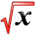# Lagrange MultiplierThis article is part of the MathHelp Tutoring Wiki

Example 1:
Question: Minimize the function f(x,y) = $1/2x^{2}-3xy+y^{2}$subject to the constrain 3x-y+1 = 0.
Solution:

1.) Use Lagrange Multiplier.
2.) Solve for y in the constrain.
3.) Minimize $1/2x^{2}-3xy+y^{2}$by using the constrain g(x,y) = 3x-y+1 = 0
4.) Let F(x,y,λ) = f(x,y) + λ(g(x,y)) = $\displaystyle (1/2)x^2-3xy+y^2+λ(3x-y+1)$
5.) F(x,y,λ) = $\displaystyle (1/2)x^2-3xy+y^2+3λx-λy+λ)$
∂F/∂x = x -3y + 3λ = 0
∂F/∂y = -3x + 2y - λ = 0
∂F/∂λ = 3x - y + 1 = 0
x - 3y = -3λ
x-3y/-3 = λ
-3x + 2y = λ
x-3y/-3 = -3x + 2y
x-3y = 9x - 6y
0 = 8x - 3y
3x + 1 = y
0 = 8x - 3(3x+1)
0 = 8x - 9x -3
0 = -x - 3
x = -3
Therefore (-3, -8) is the correct answer.

Example 2:
Question: Suppose that a units of labor and b units of capital can produce f(a,b) = 60a3/4b1/4 units of the product. Each unit of capital costs \$200. Each unit of labor costs \$100. The limit spent on production is \$30000. What is the number of units of labor and capital that should be used to maximize production?
Solution:

Constraint equation is: 100a + 200b = 30000
In other words, it is: h(a,b) = 30000 - 100x - 200y = 0.
The function we need to maximize is f(x,y) = 60a3/4b1/4.
The Lagrange Multiplier is: F(a,b,λ) = 60a3/4b1/4 + λ(30000 - 100x - 200y)1/4
∂F/∂a = 45a-1/4b1/4 - 100λ = 0
∂F/∂b = 15a3/4)b-3/4 - 200λ = 0
∂F/∂λ = 30000 - 100a - 200b = 0
Solve for λ for the first two equations and get:
λ = 45/100a-1/4b1/4 = 9/20a1/4b1/4
λ = 15/200a3/4b-3/4 = 3/40a3/4b-3/4
9/20a-1/4b1/4 = 3/40a3/4b-3/4
9/20b = 3/40a
y = 1/6a
100a + 200(1/6a) = 30000
400/3a = 30000
a = 225
b = 225/6 = 37.5
The number of units of labor is 225 and the number of units of capital is 37.5 to obtain maximum production.

Example 3:
Question: Minimize 42x + 28y, with constraint 600 - xy = 0 ( x and y are restricted to positive values.)
Solution:

Constraint equation is: 600 - xy = 0
In other words, it is: h(x,y) = 600 - xy = 0.
The function we need to minimize is f(x,y) = 42x + 28y.
The Lagrange Multiplier is: F(x,y,λ) = 42x + 28y + λ(600 - xy).
∂F/∂x = 42 - λy = 0
∂F/∂y = 28 - λx = 0
∂F/∂λ = 600 - xy = 0
Solve for λ for the first two equations and get:
λ = 42/y = 28/x
42x = 28y
x = 2/3y
600 - (2/3y)y = 0
y2= 3/2(600) = 900
y = +/- 30
y = -30 is not going to be used because we are only using positive values of x and y.
y = 30
x = 2/3(30) = 20
λ = 28/20 = 7/5
Minimum value is obtained when x = 20 and y = 30 and λ = 7/5.
The minimum value is 42(20) + 28(30) = 1680.